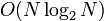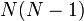# Brute-force black-box group algorithm for abelianness testing

Jump to: navigation, search

## Summary

Item Value
Problem being solved abelianness testing problem: determine whether a given group is abelian.
Input format a finite group$G$ given via an encoding of a group
Output format Yes/No depending on whether$G$ is abelian
In the No case it is also possible to output a pair of elements that do not commute.
Theoretical significance This shows that the abelianness testing problem is in$P$ by explicitly finding a polynomial-time algorithm for it.
Running time ($O(N^2)$ times the time taken for group operations) + (time taken for enumerating all group elements)
Black-box group operations used Group multiplication and an enumeration of all group elements, either as an algorithm or given explicitly. An explicit membership test is not required, though a full enumeration obviously gives a membership test as well, albeit not a very efficient one.
Competing algorithms generating set-based black-box group algorithm for abelianness testing: This is faster, but more complicated to code. It proceeds by first finding a generating set for the group using the black-box group algorithm for small generating set-finding problem, then applies the black-box group algorithm for abelianness testing given a generating set.A smarter version combines the algorithms somewhat but yields no big-oh improvement.
nondeterministic black-box group algorithm for abelianness testing: nondeterministic algorithm that first guesses a generating set, then verifies that it generates the whole group and that the elements in the generating set commute with each other. Takes time$O(N\log_2N)$ times the time taken for group operations.
randomized black-box group algorithm for abelianness testing: Takes time$O(\log_2N)$ plus the time for group operations, assuming that lookup of random elements takes logarithmic time. The idea is to randomly select pairs of elements in the group and check whether they commute. A No answer means the group is definitely non-abelian, and a Yes answer means it is probably abelian, with the trade-off between probability and the$O$-constant independent of$N$.
Parallelizable version The algorithm can be massively parallelized as long as the black-box group operations can be simultaneously accessed by multiple parallel processes. With massive parallelization, the time taken by the algorithm could be pushed down to$O(N)$, with the$O(N)$ simply representing the time costs of managing the parallel processes.

## Idea and outline

The idea is simple: for every pair$\{x,y \}$ of elements of$G$, compute the products$xy$ and$yx$ and check whether they are equal.

## Analysis of complexity

### Analysis of running time

The number of pairs is$O(N^2)$ and we assume that traversal of the pairs does not change the order of magnitude. The cost of checking each pair is twice the cost of multiplication plus the cost of equality checking. With the log-size assumption, these are all polynomial in$\log_2N$, so we get$O(N^2(\log_2N)^r)$ as the total time for the algorithm.

### Summary

Complexity measure Precise value Order (big-oh notation)
Query complexity for group multiplication i.e., number of times group multiplication is invoked, assuming an enumeration of elements is already available$N(N - 1)$ (if we skip each element with itself)$(N - 1)(N - 2)$ (if we skip all the pairs involving the identity element and all pairs of an element with itself)$O(N^2)$
Active workspace required, assuming the enumeration of elements is available on two separate one-way readable tapes enough to store finitely many group elements, and store location on tapes. All are logarithmic in$N$.$O(\log N)$